# Hyperparameter optimization with approximate gradient

Jun. 20, 2016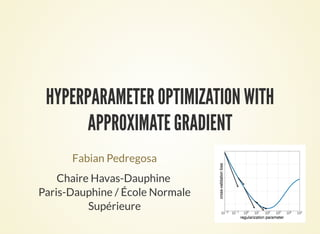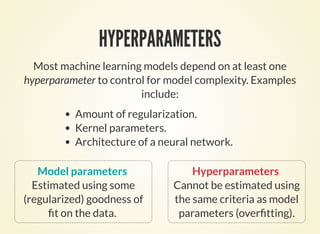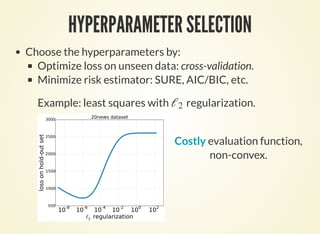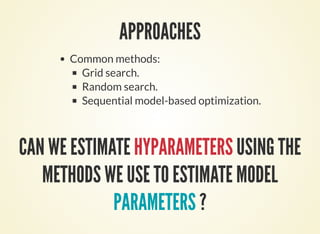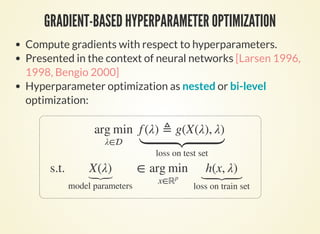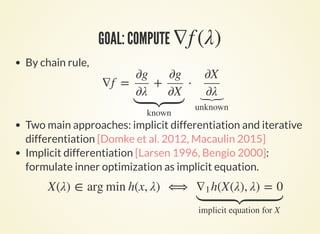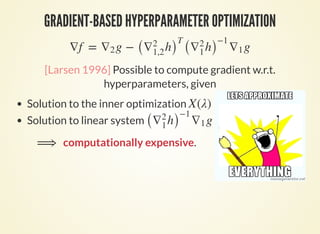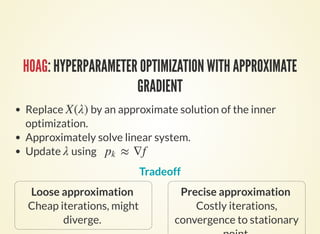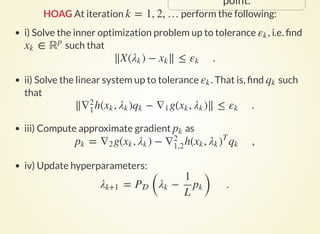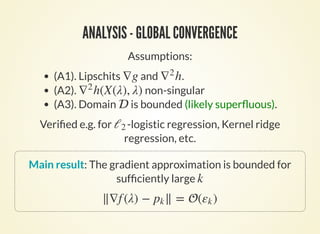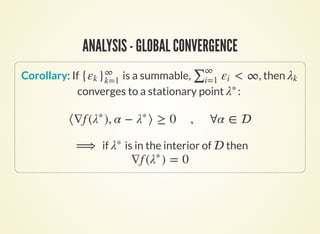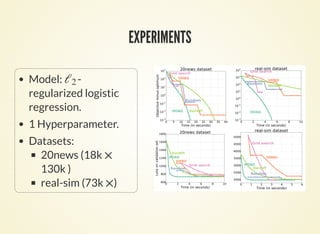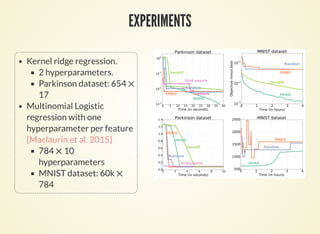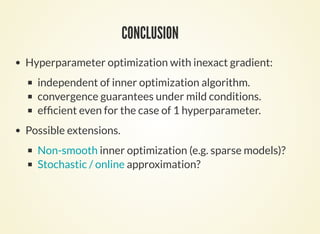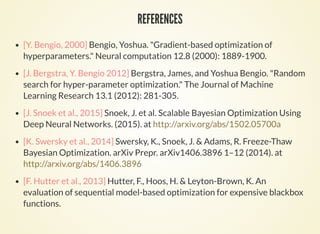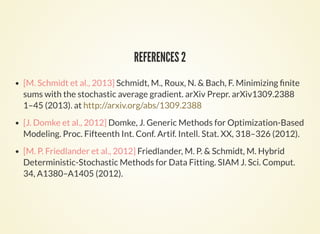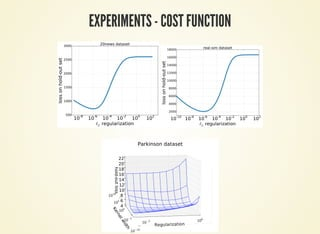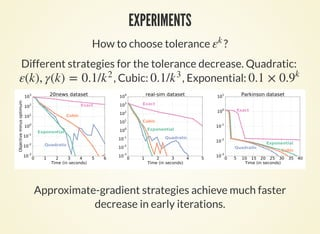1 of 18

### Hyperparameter optimization with approximate gradient

1. HYPERPARAMETER OPTIMIZATION WITH APPROXIMATE GRADIENT Fabian Pedregosa Chaire Havas-Dauphine Paris-Dauphine / École Normale Supérieure
2. HYPERPARAMETERS Most machine learning models depend on at least one hyperparameter to control for model complexity. Examples include: Amount of regularization. Kernel parameters. Architecture of a neural network. Model parameters Estimated using some (regularized) goodness of t on the data. Hyperparameters Cannot be estimated using the same criteria as model parameters (over tting).
3. HYPERPARAMETER SELECTION Criterion to for hyperparameter selection: Optimize loss on unseen data: cross-validation. Minimize risk estimator: SURE, AIC/BIC, etc. Example: least squares with regularization.ℓ2 loss = Costly evaluation function, non-convex. Common methods: grid search, random search, SMBO. ( − X(λ)∑n i=1 bi ai ) 2
4. GRADIENT-BASED HYPERPARAMETER OPTIMIZATION Compute gradients with respect to hyperparameters [Larsen 1996, 1998, Bengio 2000]. Hyperparameter optimization as nested or bi-level optimization: arg min λ∈ s.t.  X(λ) ⏟model parameters   f (λ) ≜ g(X(λ), λ)   loss on test set ∈  arg min x∈ℝp h(x, λ) ⏟loss on train set
5. GOAL: COMPUTE ∇f (λ) By chain rule, Two main approaches: implicit differentiation and iterative differentiation [Domke et al. 2012, Macaulin 2015] Implicit differentiation [Larsen 1996, Bengio 2000]: formulate inner optimization as implicit equation. ∇f = ⋅+ ∂g ∂λ ∂g ∂X   known ∂X ∂λ ⏟unknown X(λ) ∈ arg min h(x, λ) ⟺ h(X(λ), λ) = 0∇1   implicit equation for X
6. GRADIENT-BASED HYPERPARAMETER OPTIMIZATION ∇f = g − g∇2 ( h)∇2 1,2 T ( h)∇2 1 −1 ∇1 Possible to compute gradient w.r.t. hyperparameters, given Solution to the inner optimization Solution to linear system X(λ) g( h)∇2 1 −1 ∇1 computationally expensive.⟹
7. HOAG: HYPERPARAMETER OPTIMIZATION WITH APPROXIMATE GRADIENT Loose approximation Cheap iterations, might diverge. Precise approximation Costly iterations, convergence to stationary Replace by an approximate solution of the inner optimization. Approximately solve linear system. Update using Tradeoff X(λ) λ    ≈ ∇fpk
8. point. HOAG At iteration perform the following:k = 1, 2, … i) Solve the inner optimization problem up to tolerance , i.e. nd such that ii) Solve the linear system up to tolerance . That is, nd such that iii) Compute approximate gradient as iv) Update hyperparameters: εk ∈xk ℝp ∥X( ) − ∥ ≤ .λk xk εk εk qk ∥ h( , ) − g( , )∥ ≤ .∇2 1 xk λk qk ∇1 xk λk εk pk = g( , ) − h( , ,pk ∇2 xk λk ∇2 1,2 xk λk ) T qk = ( − ) .λk+1 P λk 1 L pk
9. ANALYSIS - GLOBAL CONVERGENCE Assumptions: (A1). Lipschits and . (A2). non-singular (A3). Domain is bounded. ∇g h∇2 h(X(λ), λ)∇2 1  Corollary: If , then converges to a stationary point : if is in the interior of then < ∞∑∞ i=1 εi λk λ∗ ⟨∇f ( ), α − ⟩ ≥ 0 , ∀α ∈ λ∗ λ∗ ⟹ λ∗  ∇f ( ) = 0λ∗
10. EXPERIMENTS How to choose tolerance ?εk Different strategies for the tolerance decrease. Quadratic: , Cubic: , Exponential:= 0.1/εk k 2 0.1/k 3 0.1 × 0.9 k Approximate-gradient strategies achieve much faster decrease in early iterations.
11. EXPERIMENTS I Model: -regularized logistic regression. 1 Hyperparameter. Datasets: 20news (18k 130k ) real-sim (73k 20k) ℓ2 × ×
12. EXPERIMENTS II Kernel ridge regression. 2 hyperparameters. Parkinson dataset: 654 17 Multinomial Logistic regression with one hyperparameter per feature [Maclaurin et al. 2015] 784 10 hyperparameters MNIST dataset: 60k 784 × × ×
13. CONCLUSION Hyperparameter optimization with inexact gradient: can update hyperparameters before model parameters have fully converged. independent of inner optimization algorithm. convergence guarantees under smoothness assumptions. Open questions. Non-smooth inner optimization (e.g. sparse models)? Stochastic / online approximation?
14. REFERENCES [Y. Bengio, 2000] Bengio, Yoshua. "Gradient-based optimization of hyperparameters." Neural computation 12.8 (2000): 1889-1900. [J. Bergstra, Y. Bengio 2012] Bergstra, James, and Yoshua Bengio. "Random search for hyper-parameter optimization." The Journal of Machine Learning Research 13.1 (2012): 281-305. [J. Snoek et al., 2015] Snoek, J. et al. Scalable Bayesian Optimization Using Deep Neural Networks. (2015). at [K. Swersky et al., 2014] Swersky, K., Snoek, J. & Adams, R. Freeze-Thaw Bayesian Optimization. arXiv Prepr. arXiv1406.3896 1–12 (2014). at [F. Hutter et al., 2013] Hutter, F., Hoos, H. & Leyton-Brown, K. An evaluation of sequential model-based optimization for expensive blackbox functions. http://arxiv.org/abs/1502.05700a http://arxiv.org/abs/1406.3896
15. REFERENCES 2 [M. Schmidt et al., 2013] Schmidt, M., Roux, N. & Bach, F. Minimizing nite sums with the stochastic average gradient. arXiv Prepr. arXiv1309.2388 1–45 (2013). at [J. Domke et al., 2012] Domke, J. Generic Methods for Optimization-Based Modeling. Proc. Fifteenth Int. Conf. Artif. Intell. Stat. XX, 318–326 (2012). [M. P. Friedlander et al., 2012] Friedlander, M. P. & Schmidt, M. Hybrid Deterministic-Stochastic Methods for Data Fitting. SIAM J. Sci. Comput. 34, A1380–A1405 (2012). http://arxiv.org/abs/1309.2388
16. EXPERIMENTS - COST FUNCTION
17. EXPERIMENTS Comparison with other hyperparameter optimization methods Random = Random search, SMBO = Sequential Model-Based Optimization (Gaussian process), Iterdiff = reverse-mode differentiation .
18. EXPERIMENTS Comparison in terms of a validation loss. Random = Random search, SMBO = Sequential Model-Based Optimization (Gaussian process), Iterdiff = reverse-mode differentiation .